# Big Ideas Math Geometry Chapter 8: Similarity Chapter Exam

Exam Instructions:

Choose your answers to the questions and click 'Next' to see the next set of questions. You can skip questions if you would like and come back to them later with the yellow "Go To First Skipped Question" button. When you have completed the practice exam, a green submit button will appear. Click it to see your results. Good luck!

### Page 1

#### Question 4 4. Study the given diagram. Find the value of x, the distance between T and E.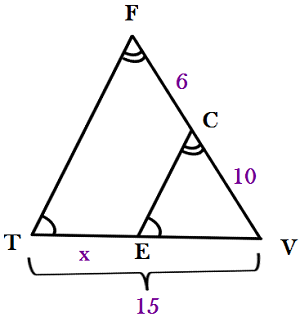#### Question 5 5. Find x.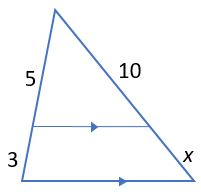### Page 2

#### Question 6 6. Given triangles DAR and KMR. What is the value of y?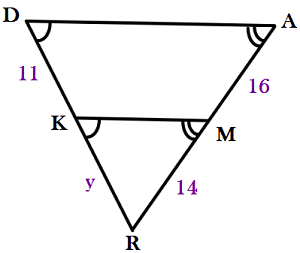#### Question 7 7. Is triangle ABC similar to triangle DEF?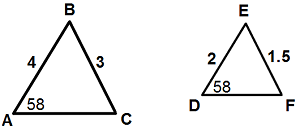#### Question 9 9. Find x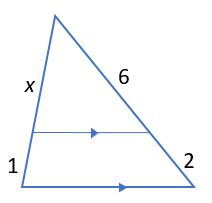### Page 3

#### Question 11 11. If BD = 1, DC = 2, AB = 2, what must AC equal for line segment AD to be an angle bisector?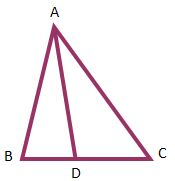#### Question 12 12. Find x.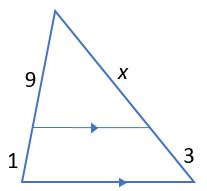#### Question 13 13. If line segment AD is an angle bisector and AB = 10, BD = 5, and DC = 7, what does AC measure?#### Question 14 14. Mary claims that the triangles below are similar by AA. Do you agree or disagree with Mary?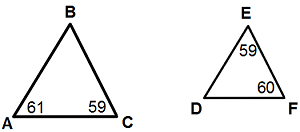### Page 4

#### Question 16 16. Which of the following is the correct ratio for the angle bisector theorem for this triangle?#### Question 17 17. Conclude whether triangle BEN is similar to triangle MAT.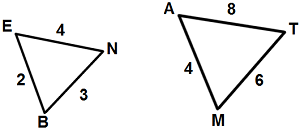#### Question 18 18. Find x.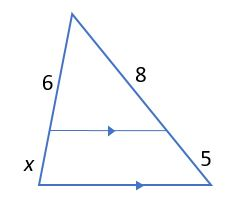#### Question 20 20. Josh claims that these triangles are similar by SSS. Do you agree or disagree with Josh?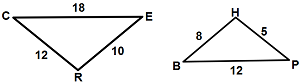### Page 5

#### Question 22 22. Determine if triangle VEZ is similar to triangle MAG.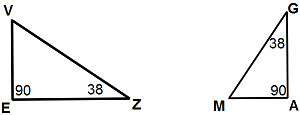#### Question 23 23. If line segment AD is an angle bisector and AB=6, AC = 8, and BD = 3, what must DC equal?#### Question 24 24. Triangle HYV and triangle AYB are similar by the AA similarity theorem. What is the value of x?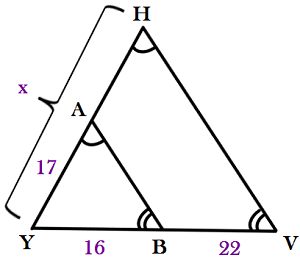#### Big Ideas Math Geometry Chapter 8: Similarity Chapter Exam Instructions

Choose your answers to the questions and click 'Next' to see the next set of questions. You can skip questions if you would like and come back to them later with the yellow "Go To First Skipped Question" button. When you have completed the practice exam, a green submit button will appear. Click it to see your results. Good luck!

Support